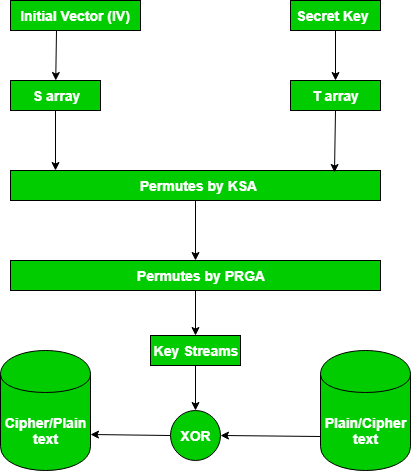Open in App
Not now

# RC4 Encryption Algorithm

• Difficulty Level : Easy
• Last Updated : 18 Oct, 2021

RC4 is a stream cipher and variable-length key algorithm. This algorithm encrypts one byte at a time (or larger units at a time).
A key input is pseudorandom bit generator that produces a stream 8-bit number that is unpredictable without knowledge of input key, The output of the generator is called key-stream, is combined one byte at a time with the plaintext stream cipher using X-OR operation.
Example:

```RC4 Encryption
10011000 ? 01010000 = 11001000

RC4 Decryption
11001000 ? 01010000 = 10011000
```

Key-Generation Algorithm –
A variable-length key from 1 to 256 bytes is used to initialize a 256-byte state vector S, with elements S to S. For encryption and decryption, a byte k is generated from S by selecting one of the 255 entries in a systematic fashion, then the entries in S are permuted again.

1. Key-Scheduling Algorithm:
Initialization: The entries of S are set equal to the values from 0 to 255 in ascending order, a temporary vector T, is created.
If the length of the key k is 256 bytes, then k is assigned to T. Otherwise, for a key with length(k-len) bytes, the first k-len elements of T as copied from K, and then K is repeated as many times as necessary to fill T. The idea is illustrated as follow:

 `for``    ``i = ``0` `to ``255` `do` `S[i] = i;``T[i] = K[i mod k - len];`

we use T to produce the initial permutation of S. Starting with S to S, and for each S[i] algorithm swap it with another byte in S according to a scheme dictated by T[i], but S will still contain values from 0 to 255 :

 `j = ``0``;``for``    ``i = ``0` `to ``255` `do``    ``{``        ``j = (j + S[i] + T[i])mod ``256``;``        ``Swap(S[i], S[j]);``    ``}`

2. Pseudo random generation algorithm (Stream Generation):
Once the vector S is initialized, the input key will not be used. In this step, for each S[i] algorithm swap it with another byte in S according to a scheme dictated by the current configuration of S. After reaching S the process continues, starting from S again

 `i, j = ``0``;``while` `(``true``)``    ``i = (i + ``1``)mod ``256``;``j = (j + S[i])mod ``256``;``Swap(S[i], S[j]);``t = (S[i] + S[j])mod ``256``;``k = S[t];`

3. Encrypt using X-Or():News:
In September 2015, Microsoft announced the end of using RC4 in Microsoft edge and internet explorer 11.

This video gives a clear example of RC4 algorithm

My Personal Notes arrow_drop_up